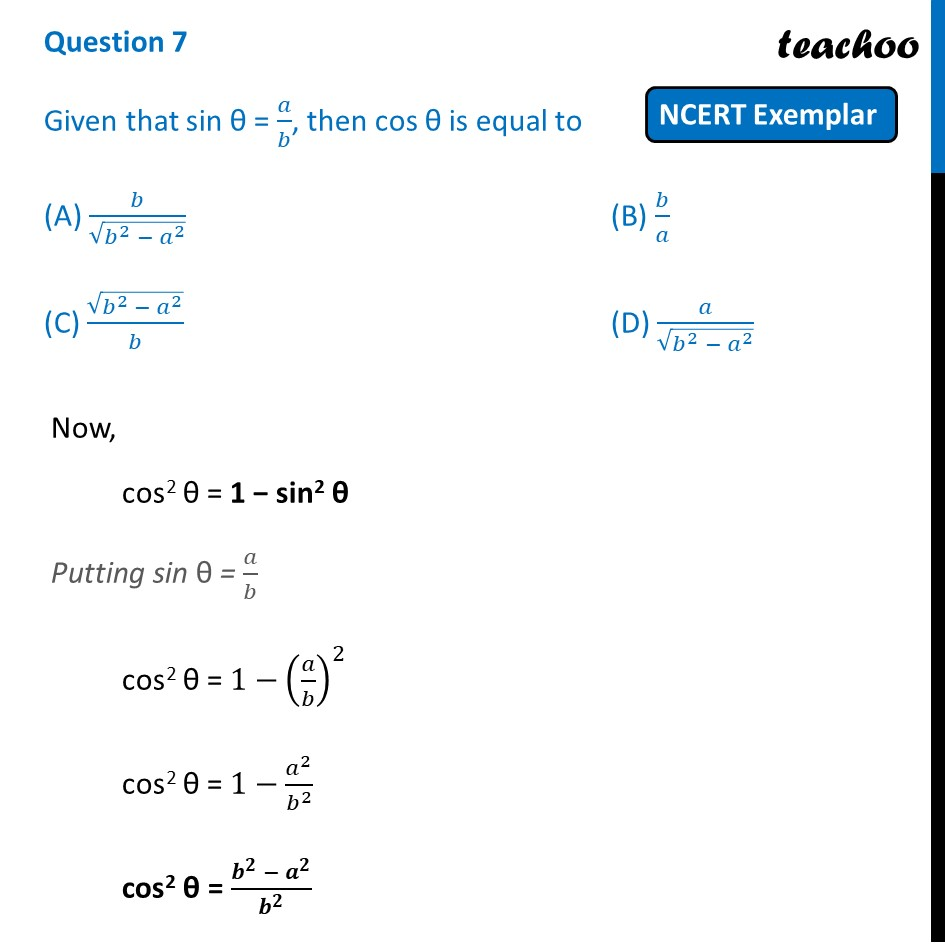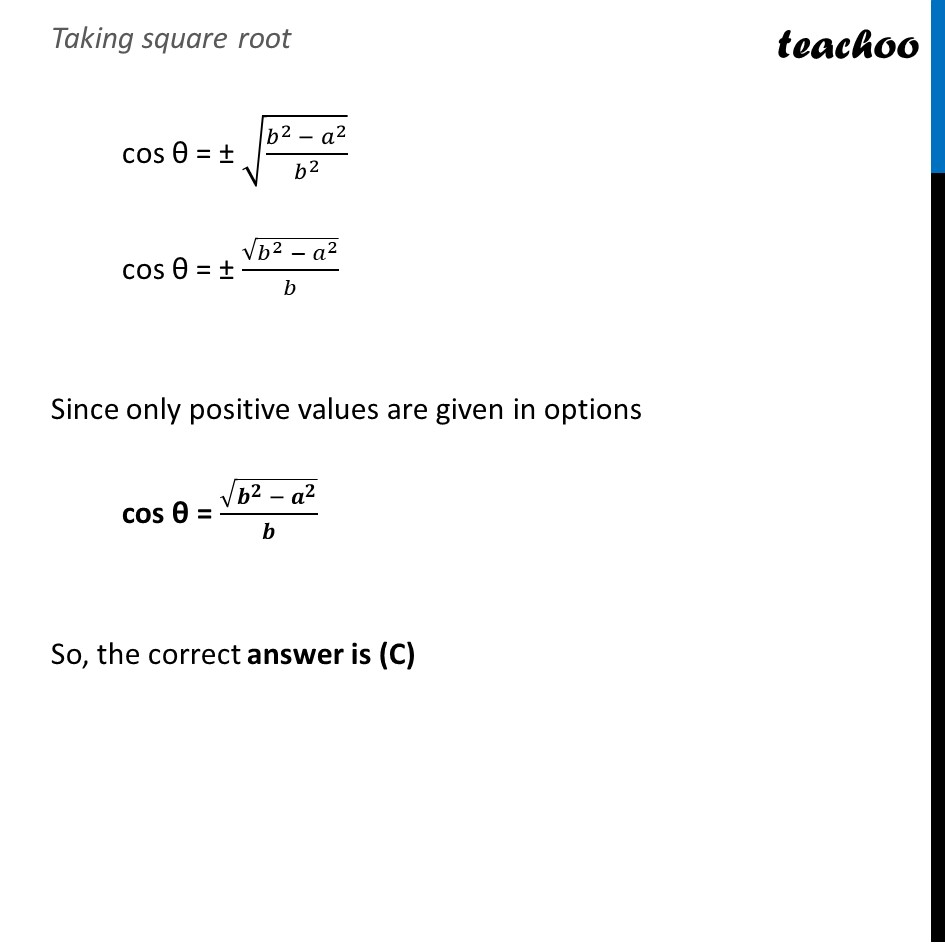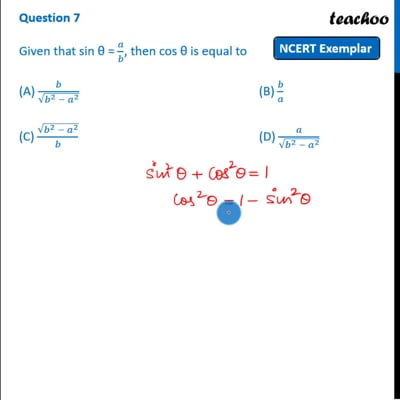NCERT Exemplar - MCQ

Chapter 8 Class 10 Introduction to Trignometry
Serial order wise

## (D) a/√(b^2  - a^2 )This video is only available for Teachoo black users

Learn in your speed, with individual attention - Teachoo Maths 1-on-1 Class

### Transcript

Question 7 Given that sin θ = 𝑎/𝑏, then cos θ is equal to (A) 𝑏/√(𝑏^2 − 𝑎^2 ) (B) 𝑏/𝑎 (C) √(𝑏^2 − 𝑎^2 )/𝑏 (D) 𝑎/√(𝑏^2 − 𝑎^2 ) Now, cos2 θ = 1 − sin2 θ Putting sin θ = 𝑎/𝑏 cos2 θ = 1−(𝑎/𝑏)^2 cos2 θ = 1−𝑎^2/𝑏^2 cos2 θ = (𝒃^𝟐 − 𝒂^𝟐)/𝒃^𝟐 Taking square root cos θ = ± √((𝑏^2 − 𝑎^2)/𝑏^2 ) cos θ = ± √(𝑏^2 − 𝑎^2 )/𝑏 Since only positive values are given in options cos θ = √(𝒃^𝟐 − 𝒂^𝟐 )/𝒃 So, the correct answer is (C)